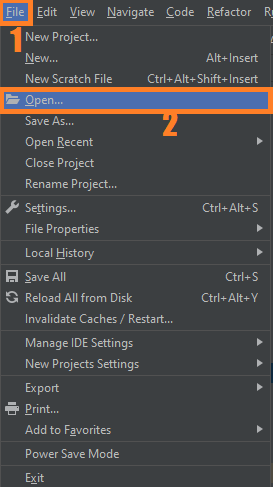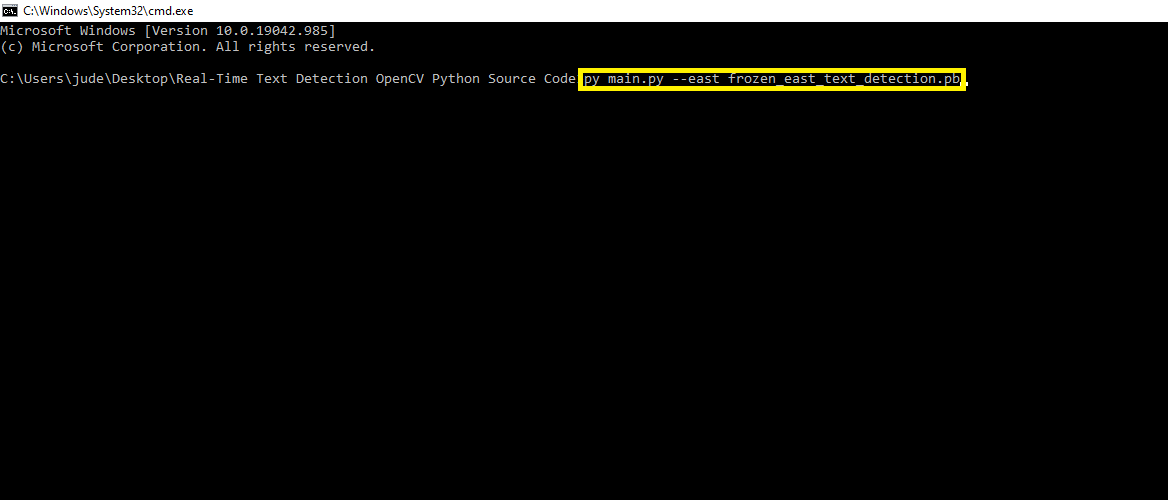# OpenCV Python East Text Detection With Source Code

The OpenCV Python Text Detection was developed using Python OpenCV, in this tutorial you will learn how to use OpenCV to detect text in real-time using web-camera.

OpenCV Python is a deep learning model, based on a novel architecture and training pattern.

In this OpenCV Text Detection Python, you will learn how to use OpenCV’s EAST detector to automatically detect text in both images and video streams.

Also, you will learn how to use OpenCV to detect text in images using the EAST text detector.

The EAST text detector requires that we are running OpenCV on our systems — if you do not already have OpenCV or better installed, please refer to my OpenCV install guides and follow the one for your respective operating system.

## Project Information

By the way, if you are new to Python programming and don’t know what Python IDE to use, I have here a list of the Best Python IDE for Windows, Linux, and Mac OS that will suit you.

To start executing Real-Time Text Detection OpenCV Python With Source Code, make sure that you have installed Python 3.9 and PyCharm on your computer.

## Steps On How To Run The Real-Time Text Detection OpenCV Python With Source Code

Time needed: 5 minutes

These are the steps on how to run Real-Time Text Detection OpenCV Python With Source Code

First, download the given source code below and unzip the source code.• Step 2: Import the project to your PyCharm IDE.• Step 3: Run the project.

Lastly, run the project with the command “py main.py”## Installed Libraries

```from imutils.video import VideoStream
from imutils.video import FPS
from imutils.object_detection import non_max_suppression
import numpy as np
import argparse
import imutils
import time
import cv2```

## Complete Source Code

```# USAGE
# python text_detection_video.py --east frozen_east_text_detection.pb

# import the necessary packages
from imutils.video import VideoStream
from imutils.video import FPS
from imutils.object_detection import non_max_suppression
import numpy as np
import argparse
import imutils
import time
import cv2

def decode_predictions(scores, geometry):
# grab the number of rows and columns from the scores volume, then
# initialize our set of bounding box rectangles and corresponding
# confidence scores
(numRows, numCols) = scores.shape[2:4]
rects = []
confidences = []

# loop over the number of rows
for y in range(0, numRows):
# extract the scores (probabilities), followed by the
# geometrical data used to derive potential bounding box
# coordinates that surround text
scoresData = scores[0, 0, y]
xData0 = geometry[0, 0, y]
xData1 = geometry[0, 1, y]
xData2 = geometry[0, 2, y]
xData3 = geometry[0, 3, y]
anglesData = geometry[0, 4, y]

# loop over the number of columns
for x in range(0, numCols):
# if our score does not have sufficient probability,
# ignore it
if scoresData[x] < args["min_confidence"]:
continue

# compute the offset factor as our resulting feature
# maps will be 4x smaller than the input image
(offsetX, offsetY) = (x * 4.0, y * 4.0)

# extract the rotation angle for the prediction and
# then compute the sin and cosine
angle = anglesData[x]
cos = np.cos(angle)
sin = np.sin(angle)

# use the geometry volume to derive the width and height
# of the bounding box
h = xData0[x] + xData2[x]
w = xData1[x] + xData3[x]

# compute both the starting and ending (x, y)-coordinates
# for the text prediction bounding box
endX = int(offsetX + (cos * xData1[x]) + (sin * xData2[x]))
endY = int(offsetY - (sin * xData1[x]) + (cos * xData2[x]))
startX = int(endX - w)
startY = int(endY - h)

# add the bounding box coordinates and probability score
# to our respective lists
rects.append((startX, startY, endX, endY))
confidences.append(scoresData[x])

# return a tuple of the bounding boxes and associated confidences
return (rects, confidences)

# construct the argument parser and parse the arguments
ap = argparse.ArgumentParser()
help="path to input EAST text detector")
help="path to optinal input video file")
help="minimum probability required to inspect a region")
help="resized image width (should be multiple of 32)")
help="resized image height (should be multiple of 32)")
args = vars(ap.parse_args())

# initialize the original frame dimensions, new frame dimensions,
# and ratio between the dimensions
(W, H) = (None, None)
(newW, newH) = (args["width"], args["height"])
(rW, rH) = (None, None)

# define the two output layer names for the EAST detector model that
# we are interested -- the first is the output probabilities and the
# second can be used to derive the bounding box coordinates of text
layerNames = [
"feature_fusion/Conv_7/Sigmoid",
"feature_fusion/concat_3"]

# load the pre-trained EAST text detector

# if a video path was not supplied, grab the reference to the web cam
if not args.get("video", False):
print("[INFO] starting video stream...")
vs = VideoStream(src=0).start()
time.sleep(1.0)

# otherwise, grab a reference to the video file
else:
vs = cv2.VideoCapture(args["video"])

# start the FPS throughput estimator
fps = FPS().start()

# loop over frames from the video stream
while True:
# grab the current frame, then handle if we are using a
# VideoStream or VideoCapture object
frame = frame if args.get("video", False) else frame

# check to see if we have reached the end of the stream
if frame is None:
break

# resize the frame, maintaining the aspect ratio
frame = imutils.resize(frame, width=1000)
orig = frame.copy()

# if our frame dimensions are None, we still need to compute the
# ratio of old frame dimensions to new frame dimensions
if W is None or H is None:
(H, W) = frame.shape[:2]
rW = W / float(newW)
rH = H / float(newH)

# resize the frame, this time ignoring aspect ratio
frame = cv2.resize(frame, (newW, newH))

# construct a blob from the frame and then perform a forward pass
# of the model to obtain the two output layer sets
blob = cv2.dnn.blobFromImage(frame, 1.0, (newW, newH),
(123.68, 116.78, 103.94), swapRB=True, crop=False)
net.setInput(blob)
(scores, geometry) = net.forward(layerNames)

# decode the predictions, then  apply non-maxima suppression to
# suppress weak, overlapping bounding boxes
(rects, confidences) = decode_predictions(scores, geometry)
boxes = non_max_suppression(np.array(rects), probs=confidences)

# loop over the bounding boxes
for (startX, startY, endX, endY) in boxes:
# scale the bounding box coordinates based on the respective
# ratios
startX = int(startX * rW)
startY = int(startY * rH)
endX = int(endX * rW)
endY = int(endY * rH)

# draw the bounding box on the frame
cv2.rectangle(orig, (startX, startY), (endX, endY), (0, 255, 0), 2)

# update the FPS counter
fps.update()

# show the output frame
cv2.imshow("Text Detection", orig)
key = cv2.waitKey(1) & 0xFF

# if the `q` key was pressed, break from the loop
if key == ord("q"):
break

# stop the timer and display FPS information
fps.stop()
print("[INFO] elasped time: {:.2f}".format(fps.elapsed()))
print("[INFO] approx. FPS: {:.2f}".format(fps.fps()))

# if we are using a webcam, release the pointer
if not args.get("video", False):
vs.stop()

# otherwise, release the file pointer
else:
vs.release()

# close all windows
cv2.destroyAllWindows()```

## Summary

In this article, Text detection deals with detecting the presence of the text in the input image or real-time, whereas text localization localizes the position of the text and forms groups of text regions by eliminating the maximum of the background.

Text detection and localization process is performed using connected component analysis or region-based methods.

## Inquiries

### 3 thoughts on “OpenCV Python East Text Detection With Source Code”

1.Hi sir,
Myself Nayana R
I have referred your source code for my project .So can I get report regarding text detection project . If you send the report it may be very much helpful to us .So ,that I can perform better in my seminars that will help me alot .

2.Hi sir ,
Myself Nayana R from Bangalore
I have referred Your project source code of text detection .So can I get the report regarding to this project ,it will helpful to me alot.

•So far we don’t have a report for this project